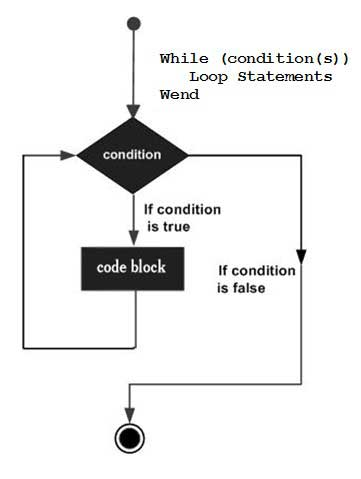# VBScript While...Wend Loop

In a While..Wend loop, if the condition is True, all statements are executed until Wend keyword is encountered.

If the condition is false, the loop is exited and the control jumps to very next statement after Wend keyword.

## Syntax

The syntax of a While..Wend loop in VBScript is −

```While condition(s)
[statements 1]
[statements 2]
...
[statements n]
Wend
```

## Flow Diagram## Example

```<!DOCTYPE html>
<html>
<body>
<script language = "vbscript" type = "text/vbscript">
Dim Counter :  Counter = 10
While Counter < 15    ' Test value of Counter.
Counter = Counter + 1   ' Increment Counter.
document.write("The Current Value of the Counter is : " & Counter)
document.write("<br></br>")
Wend ' While loop exits if Counter Value becomes 15.

</script>
</body>
</html>
```

When the above code is executed, it prints the following output in the console.

```The Current Value of the Counter is : 11

The Current Value of the Counter is : 12

The Current Value of the Counter is : 13

The Current Value of the Counter is : 14

The Current Value of the Counter is : 15
```
vbscript_loops.htm